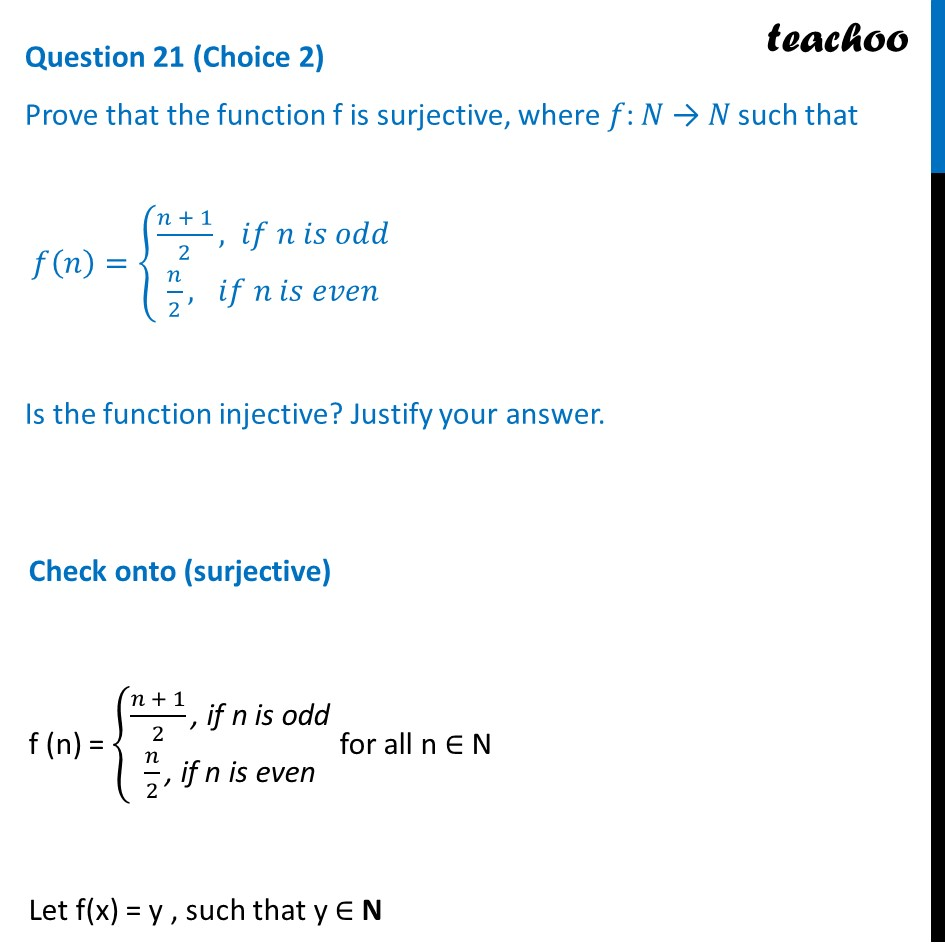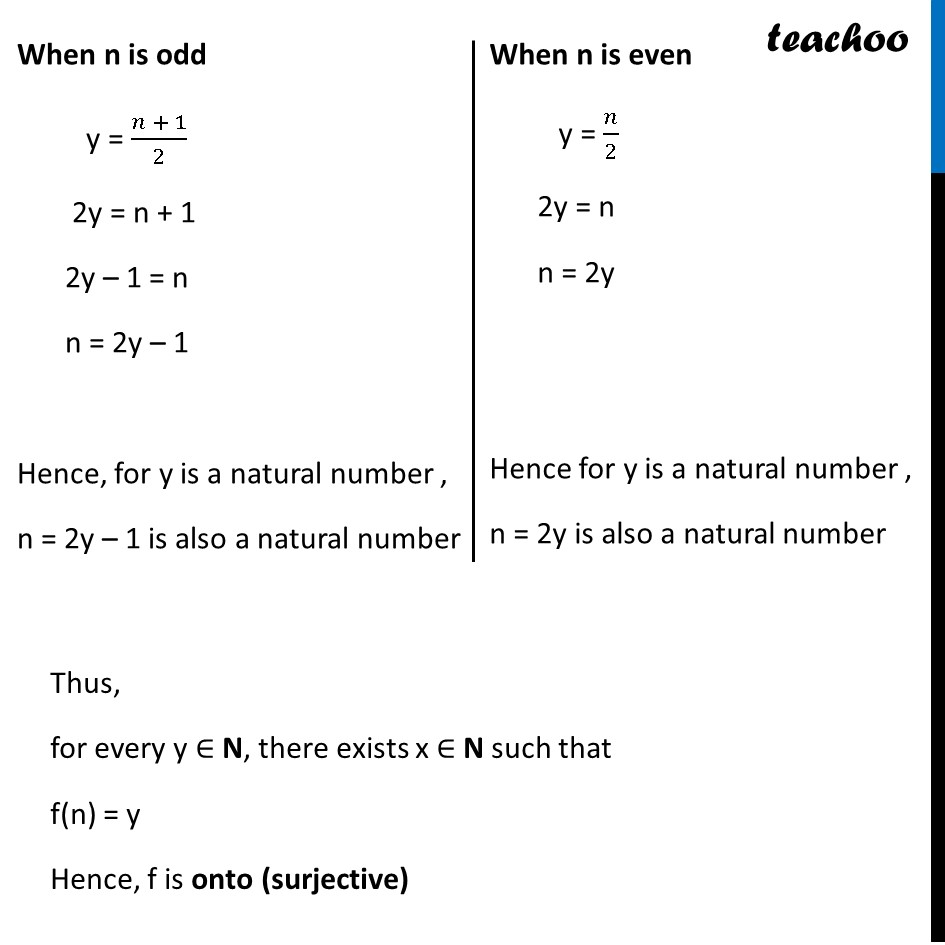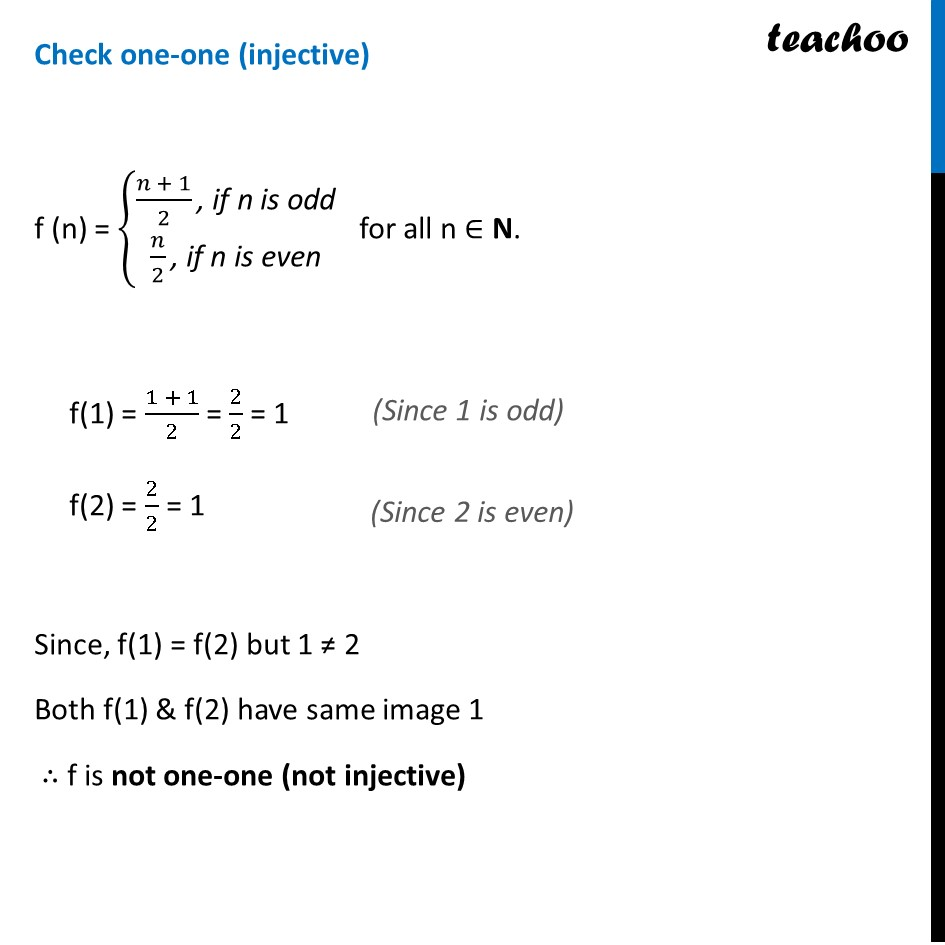CBSE Class 12 Sample Paper for 2023 Boards

Class 12
Solutions of Sample Papers and Past Year Papers - for Class 12 Boards

## f(n)={(n + 1)/2,  if n is odd n/2,  if n is evenLearn in your speed, with individual attention - Teachoo Maths 1-on-1 Class

### Transcript

Question 21 (Choice 2) Prove that the function f is surjective, where 𝑓: 𝑁 → 𝑁 such that 𝑓(𝑛)={█((𝑛 + 1)/2, 𝑖𝑓 𝑛 𝑖𝑠 𝑜𝑑𝑑@&𝑛/2, 𝑖𝑓 𝑛 𝑖𝑠 𝑒𝑣𝑒𝑛)┤ Is the function injective? Justify your answer. Check onto (surjective) f (n) = {█((𝑛 + 1)/2 ", if n is odd" @𝑛/2 ", if n is even" )┤ for all n ∈ N Let f(x) = y , such that y ∈ N When n is odd y = (𝑛 + 1)/2 2y = n + 1 2y – 1 = n n = 2y – 1 Hence, for y is a natural number , n = 2y – 1 is also a natural number When n is even y = 𝑛/2 2y = n n = 2y Hence for y is a natural number , n = 2y is also a natural number Thus, for every y ∈ N, there exists x ∈ N such that f(n) = y Hence, f is onto (surjective) Check one-one (injective) f (n) = {█((𝑛 + 1)/2 ", if n is odd" @𝑛/2 ", if n is even" )┤ for all n ∈ N. f(1) = (1 + 1)/2 = 2/2 = 1 f(2) = 2/2 = 1 Since, f(1) = f(2) but 1 ≠ 2 Both f(1) & f(2) have same image 1 ∴ f is not one-one (not injective)